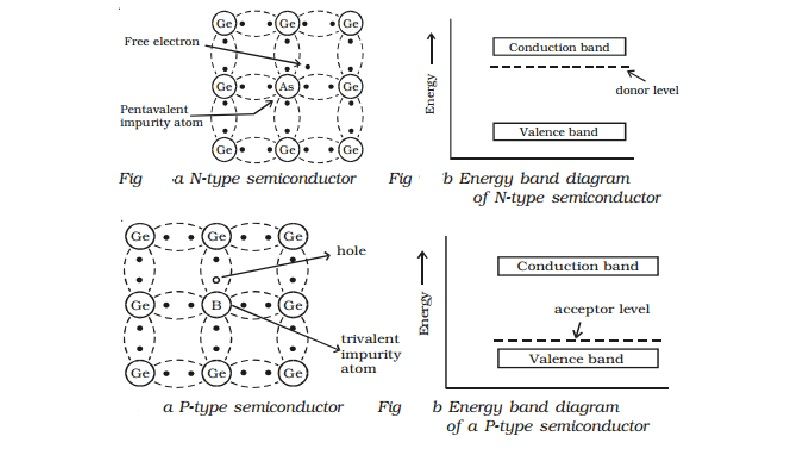Home | | Physics | | Physics | Extrinsic semiconductor: N and P -type semiconductor

# Extrinsic semiconductor: N and P -type semiconductorAn extrinsic semiconductor is one in which an impurity with a valency higher or lower than the valency of the pure semiconductor is added, so as to increase the electrical conductivity of the semiconductor.

Extrinsic semiconductor

An extrinsic semiconductor is one in which an impurity with a valency higher or lower than the valency of the pure semiconductor is added, so as to increase the electrical conductivity of the semiconductor.

Depending upon the type of impurity atoms added, an extrinsic semiconductor can be classified as N-type or P-type.

(a) N-type semiconductor

When a small amount of pentavalent impurity such as arsenic is added to a pure germanium semiconductor crystal, the resulting crystal is called N-type semiconductor.Fig a shows the crystal structure obtained when pentavalent arsenic impurity is added with pure germanium crystal. The four valence electrons of arsenic atom form covalent bonds with electrons of neighbouring four germanium atoms. The fifth electron of arsenic atom is loosely bound. This electron can move about almost as freely as an electron in a conductor and hence it will be the carrier of current. In the energy band picture, the energy state corresponding to the fifth valence electron is in the forbidden gap and lies slightly below the conduction band (Figb). This level is known as the donor level.

When the fifth valence electron is transferred to the conduction band, the arsenic atom becomes positively charged immobile ion. Each impurity atom donates one free electron to the semiconductor. These impurity atoms are called donors.

In N-type semiconductor material, the number of electrons increases, compared to the available number of charge carriers in the intrinsic semiconductor. This is because, the available larger number of electrons increases the rate of recombination of electrons with holes. Hence, in N-type semiconductor, free electrons are the majority charge carriers and holes are the minority charge carriers.

(b) P-type semiconductor

When a small amount of trivalent impurity (such as indium, boron or gallium) is added to a pure semiconductor crystal, the resulting semiconductor crystal is called P-type semiconductor.Fig a. shows the crystal structure obtained, when trivalent boron impurity is added with pure germanium crystal. The three valence electrons of the boron atom form covalent bonds with valence electrons of three neighbourhood germanium atoms. In the fourth covalent bond, only one valence electron is available from germanium atom and there is deficiency of one electron which is called as a hole. Hence for each boron atom added, one hole is created. Since the holes can accept electrons from neighbourhood, the impurity is called acceptor. The hole, may be filled by the electron from a neighbouring atom, creating a hole in that position from where the electron moves. This process continues and the hole moves about in a random manner due to thermal effects. Since the hole is associated with a positive charge moving from one position to another, this is called as P-type semiconductor. In the P-type semiconductor, the acceptor impurity produces an energy level just above the valence band. (Fig b). Since, the energy difference between acceptor energy level and the valence band is much smaller, electrons from the valence band can easily jump into the acceptor level by thermal agitation.

In P-type semiconductors, holes are the majority charge carriers and free electrons are the minority charge carriers.

Study Material, Lecturing Notes, Assignment, Reference, Wiki description explanation, brief detail

Related Topics